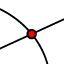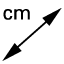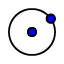# Circumcenter - 2019

Topic:
Geometry

## Follow the steps below to construct the circumcenter.

Useto construct the perpendicular bisector of segments AB, BC and AC. Useto add a point where the three perpendicular bisectors intersect. Useto label the point where the perpendicular bisectors intersect.

The point where all three perpendicular bisectors intersect is called the circumcenter. 1. Useto move point A, B or C so that the circumcenter is outside the triangle. What do you notice about the triangle?

Check all that apply

2. Useto move point C so that the circumcenter lies on a side of the triangle. What do you notice about the triangle?

Check all that apply

## We are now going to learn about the properties of a circumcenter.

Useto draw a segment from A to the circumcenter. Useto measure the length of the segment from A to the circumcenter. Repeat the process for vertex B and C Useto draw a circle with center at the circumcenter and through point A.

## Circumcenter Properties

3. Useto move point A, B or C around. What do you notice about the dashed segment lengths?

## The Circle

4. You constructed the circle to go through point A. When you move the triangle, you should notice that the circle also always goes through B and C. Why is that?

## Application for Circumcenter

5. For which of the following situations, would it make sense to find the circumcenter?

Check all that apply